RS Aggarwal Class 8 Solutions Chapter 15 - Quadrilaterals

RS Aggarwal Class 8 Chapter 15 - Quadrilaterals Solutions Free PDF

RS Aggarwal class 8 solutions chapter 15 quadrilaterals is provided here. In Euclidean Geometry a quadrilateral is a polygon with four sides. Quad means four and lateral means side. Learn and practice the concepts of quadrilaterals from RS Aggarwal for class 8. Learning the concepts of quadrilaterals will also help the students to understand the concepts of geometry and class 8 as well as in higher classes.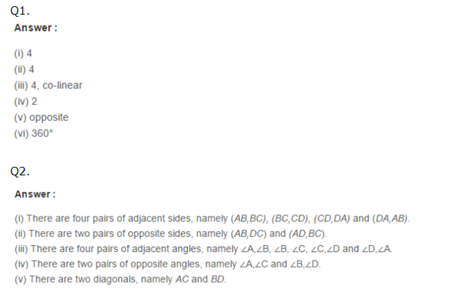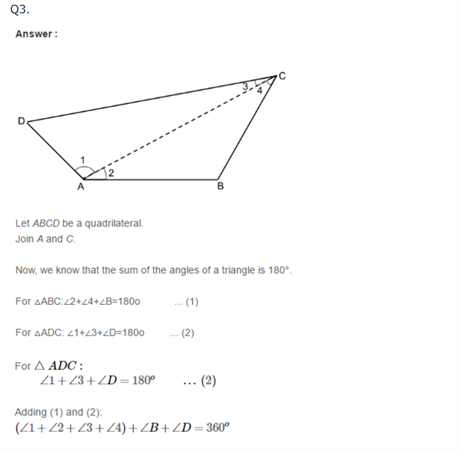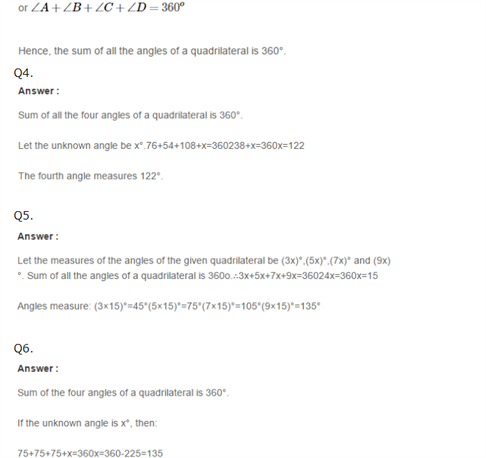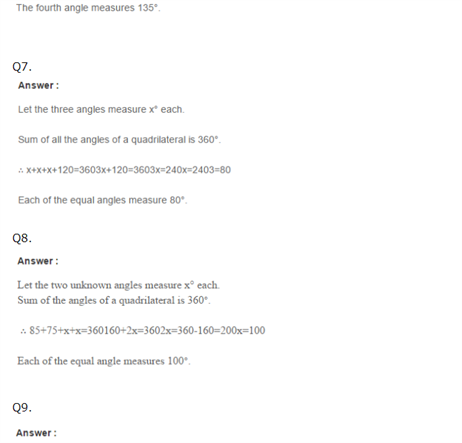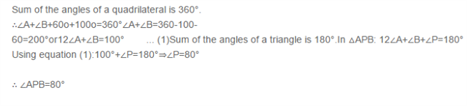Practise This Question

What are the bright objects that are in the night sky which are not luminous?